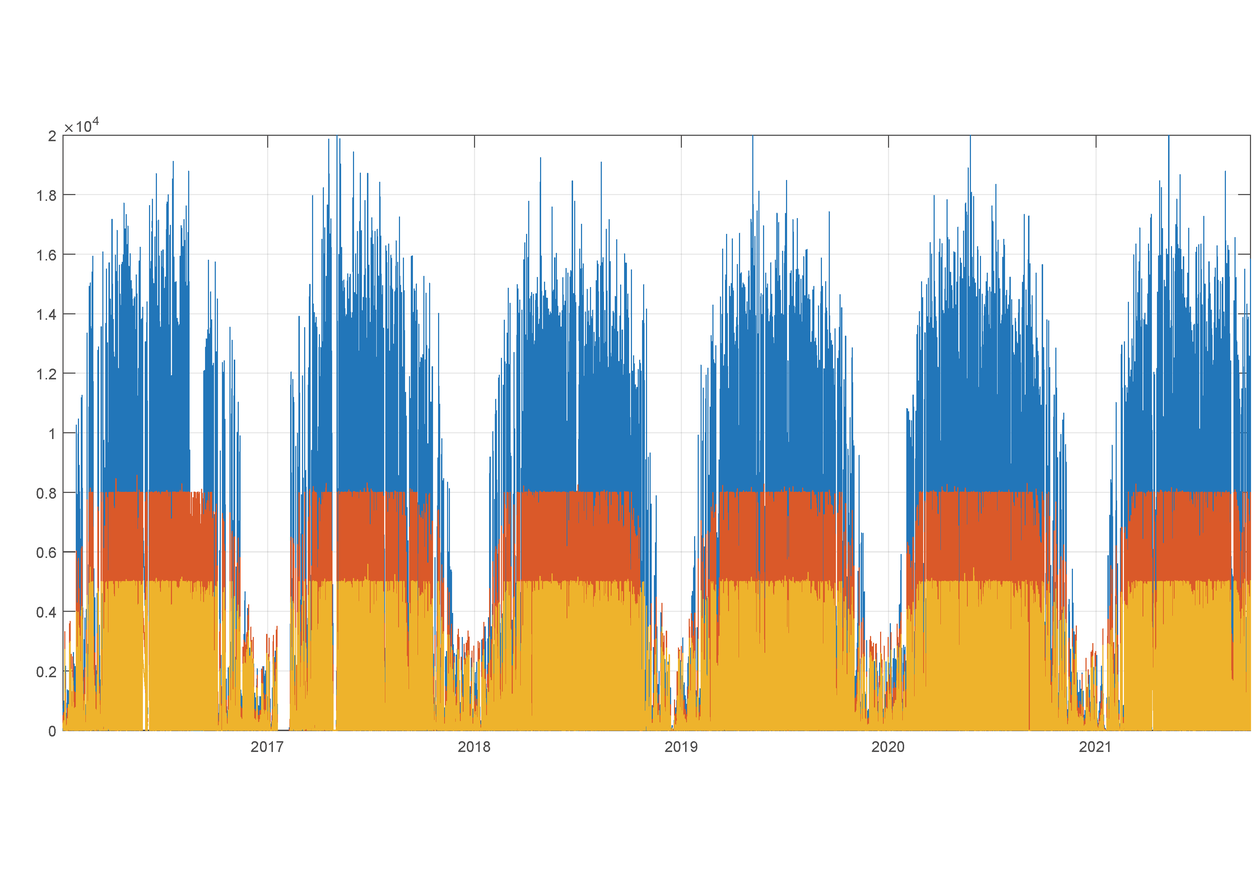Long-term measurements from a photovoltaic power plant and meteorological stations at the Laboratory LINTE^2 of Gdańsk University of Technology - Open Research Data - MOST Wiedzy

Long-term measurements from a photovoltaic power plant and meteorological stations at the Laboratory LINTE^2 of Gdańsk University of TechnologyDescription

1)    The Laboratory LINTE^2 is a large research infrastructure operated by the Faculty of Electrical and Control Engineering at Gdańsk University of Technology, to be found in Gdańsk, Poland (54.3690 N, 18.6130 E).
2)    The measurements have been collected from a photovoltaic power plant located on the roof of the laboratory, having total capacity of 33 kWp. Electrical data have been gathered from three PV inverters (SMA Sunny Tripower type, indicated in the dataset by the suffixes: _inv#1, _inv#2, _inv#3), while data of weather conditions have been gathered from two meteorological stations (SMA Weather Station type, indicated in the dataset by the suffixes: _met#1, _met#2).
3)    Raw measurements have been collected in the SCADA system of the laboratory. The dataset has been prepared using a script in MATLAB software that has converted data from the SCADA data flies into CSV file(s). The script has also eliminated incorrect data (indicated as NaN ) that might have happened due to failures of power plant devices or the SCADA system.
4)    Date range of the dataset is from 1 Jan 2016 till 30 Sep 2021  (further additions of data are foreseen). The name of the file in the dataset indicates the date range of the dataset, using the following date format for the start and the end dates: yyyymmdd.
5)    Time step of the measurements is 30 minutes.
6)    The dataset is organized as follows:

 Column in the CSV file Name of the measured data (column header in the CSV fie) Description A Date and time Format: month/day/year  hour:minute:second AM/PM B P_AC_inv#1_W Total active power in all phases of pv inverter #1 (20 kWp) expressed in watts C P_AC_inv#2_W Total active power in all phases of pv inverter #2 (8 kWp) expressed in watts D P_AC_inv#3_W Total active power in all phases of pv inverter #3 (5 kWp) expressed in watts E Q_AC_inv#1_var Total reactive power in all phases of pv inverter #1 (20 kWp) expressed in vars F Q_AC_inv#2_var Total reactive power in all phases of pv inverter #2 (8 kWp) expressed in vars G Q_AC_inv#3_var Total reactive power in all phases of pv inverter #3 (5 kWp) expressed in vars H P_DC_inv#1_W DC input power of pv inverter #1 (20 kWp) expressed in watts I P_DC_inv#2_W DC input power of pv inverter #2 (8 kWp) expressed in watts J P_DC_inv#3_W DC input power of pv inverter #3 (5 kWp) expressed in watts K I_DC_inv#1_A DC input current of pv inverter #1 (20 kWp) expressed in amperes L I_DC_inv#2_A DC input current of pv inverter #2 (8 kWp) expressed in amperes M I_DC_inv#3_A DC input current of pv inverter #3 (5 kWp) expressed in amperes N U_DC_inv#1_V DC input voltage of pv inverter #1 (20 kWp) expressed in volts O U_DC_inv#2_V DC input voltage of pv inverter #2 (8 kWp) expressed in volts P U_DC_inv#3_V DC input voltage of pv inverter #3 (5 kWp) expressed in volts Q E_sum_inv#1_kWh Total electricity generated by pv inverter #1 (20 kWp) expressed in kilowatt-hours R E_sum_inv#2_kWh Total electricity generated by pv inverter #2 (8 kWp) expressed in kilowatt-hours S E_sum_inv#3_kWh Total electricity generated by pv inverter #3 (5 kWp) expressed in kilowatt-hours T Solar_rad_met#1_W_sq_m Solar radiation intensity measured by meteorological station #1 expressed in watts per square meter U Solar_rad_met#2_W_sq_m Solar radiation intensity measured by meteorological station #2 expressed in watts per square meter V Env_temp_met#1_C Ambient temperature measured by meteorological station #1 expressed in degrees Celsius W Env_temp_met#2_C Ambient temperature measured by meteorological station #2 expressed in degrees Celsius X Panel_temp_met#1_C PV panel temperature measured by meteorological station #1 expressed in degrees Celsius Y Panel_temp_met#2_C PV panel temperature measured by meteorological station #2 expressed in degrees Celsius Z Rel_humid_met#1_perc Relative humidity of air measured by meteorological station #1 expressed in percent AA Rel_humid_met#2_perc Relative humidity of air measured by meteorological station #2 expressed in percent AB Atm_pres_met#1_hPa Atmospheric pressure measured by meteorological station #1 expressed in hectopascals AC Atm_pres_met#2_hPa Atmospheric pressure measured by meteorological station #2 expressed in hectopascalsDataset file

pv_meteo_meas_data_20160101-20210930_ORD.zip

Details

Year of publication:
2021
Verification date:
2021-12-21
Dataset language:
English
Fields of science:
• Automation, electronic and electrical engineering (Engineering and Technology)
DOI:
DOI ID 10.34808/06j6-gt25 open in new tab
Verified by:
Gdańsk University of Technology

seen 18 times